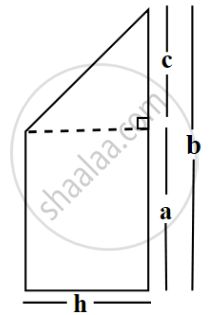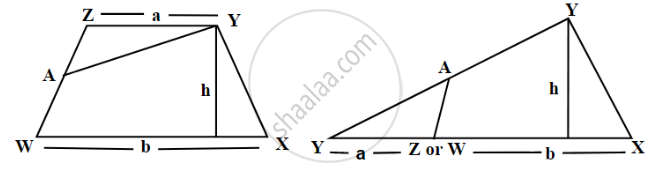# Area of Trapezium

## Formula

Area of the trapezium = 1/2 × sum of the lengths of parallel sides × height.

# Area of Trapezium:

A trapezium is a 2d shape and a type of quadrilateral, which has only two parallel sides and the other two sides are non-parallel.

In the area of a trapezium, we need to know the length of the parallel sides and the perpendicular distance between these two parallel sides. Half the product of the sum of the lengths of parallel sides and the perpendicular distance between them gives the area of trapezium.Area of triangle (∆) = 1/2 × h × c.

Area of rectangle (□) = h × a

Area of trapezium = Area of triangle(∆) + Area of rectangle (□)

We can write the area by combining the two areas and write the area of trapezium as

area of trapezium = 1/2 × h × c + h × a

area of trapezium = h(c/2 + a)

area of trapezium = h((c + 2a)/2)

area of trapezium = h((c + a + a)/2)

area of trapezium = h((b + a)/2)

area of trapezium = "height" ("Sum of parallel sides")/2

By substituting the values of h, b, and a in this expression, we find h((b + a)/2).

#### Area of Trapezium by Finding the Area of a Triangle of Same Area:

The area of the trapezium can be found out by dividing it into three parts.Draw trapezium WXYZ.

Find the midpoint of WZ by folding the side and name it A.

Cut trapezium WXYZ into two pieces by cutting along AY.

Place ∆YZA as shown in the Figure, where AZ is placed on AW.

The area of this triangle and the area of the trapezium WXYZ are same.

We know that the Area of a triangle = 1/2 × base × height

Substituting the values,

Area of a triangle = 1/2 × (a + b) × h

Area of trapezium = h((b + a)/2)

Area of a trapezium = half of the sum of the lengths of parallel sides × perpendicular distance between them.

If you would like to contribute notes or other learning material, please submit them using the button below.

### Shaalaa.com

Area of a Trapezium [00:17:58]
S
0%